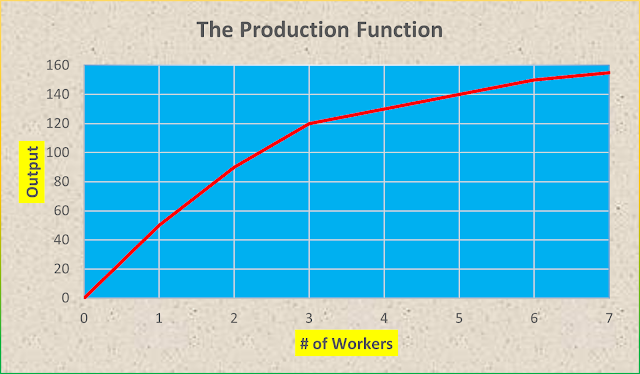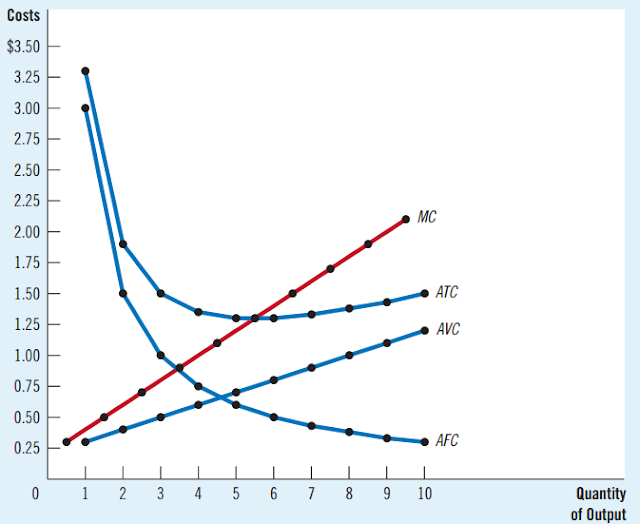# Cost of Production

## What is the cost of production?

Cost of Production is the value of money used in production.

Cost is further divided into two explicit costs and Implicit costs.

Explicit cost Require an outlay of money e.g., paying wages to workers. Whereas, Implicit

costs do not require a cash outlay e.g., the opportunity cost of the owner’s time.

Total cost = Explicit Costs + Implicit costs

Total Revenue (TR) is the amount a firm receives to sell its output.

TR = P.Q

Total Cost (TC) is the market value of the inputs a firm uses in production.

TC = ATC.Q

Profit is total revenue minus total cost.

Profit = TR - TC

Accounting Profit = TR - Explicit Cost

Economic Profit = TR - (Explicit cost + Implicit cost)

Due to the law of diminishing marginal product, output increases as the number of workers increases output, but the output is increasing at a decreasing rate.Cost of Production

Notice that as the number of workers is increasing, marginal product declines, and this property is known as diminishing marginal product.

Due to the law of diminishing marginal product, as output increases due to an increase in total cost, the output is increasing at a decreasing rate.

In the short-run fixed cost remain fixed e.g., equipment shared by the workers remain

constant as the number of workers increases. Therefore, more workers

share set equipment and space in the short run.

The Various Measures of Cost

Average total cost (ATC) is total cost divided by the quantity of output

Average fixed cost (AFC) is fixed cost divided by the quantity of output

Average variable cost (AVC) variable cost divided by the quantity of output

Marginal cost (MC) is the increase in total cost that arises from an extra unit of production.

The following figure shows the average total cost (ATC), average fixed cost (AFC),

average variable cost (AVC), and marginal cost (MC) for firm “X’. These cost curves show

three common features:

The marginal cost rises with the quantity of output.

The average-total-cost curve is U-shaped.

The marginal-cost curve crosses the average-total-cost curve at the minimum of the average total cost.Relationship MC ATC AVC & AFS

coin :  0